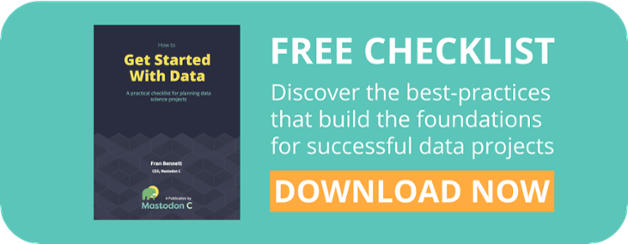Data exploration is perhaps one of the most important elements when designing a data science project. Exploring the data allows you to better plan your project and answer important questions – like whether your data can actually answer the questions you’re trying to answer. In this short guide we outline how we approach investigating a new dataset, step by step. Typically, with a new data science project you will be working with a new dataset. Investigation of the data (it’s content and composition), will help to inform how the project should proceed and whether the data can answer the question you are aiming to address.

These are crucial first steps in your project and in this blog we approach it from a data exploration angle. Whilst some of the terms may seem quite technical, most people should be able to follow how it all fits together, and pick up insights into how data exploration works in practice. If you’re looking for a more general perspective, or for more information on shaping and designing a data science project, see our Get Started with Data Guide.Follow these four steps for effective data exploration.

## step 1: divide data into response and explanatory variables

The first step is to categorise the data you are working with into “response” and “explanatory” variables. We use explanatory variables to account for variation in the response variable, and this will allow us to begin to test how our variables are related. A simple example of a response and explanatory variable, can be seen in terms of the speed and distance a car travels. We can make changes to the speed the car travels (the explanatory variable), to exhibit changes we see in the distance travelled (response). The distance travelled, however, has no influence on the speed.

The first step therefore is to distinguish your data into the two types of variables.

• Response variable (you may be more used to other terms for this e.g. Y-variable or dependent variable). This is something we can’t directly control, often considered the output of changes to the explanatory variable.
• Explanatory variable/s (you may be more used to other terms for this e.g. X-variable or independent variable). This is the variable we are able to change and model, in order to influence changes upon the response variable.

## step 2: define your explanatory variables

Categorising your variables allows you to work out what sort of mathematical models you could apply to your dataset. You need to distinguish whether the explanatory variables (the variables that account for variation in the response variable) are categorical or continuous, and identify the levels of categorical variables, crudely:

• many different numerical values = continuous (or covariate)
• non-numerical values = categorical (or factor)

An example of a continuous explanatory variable might be mean daily temperature. Theoretically this could be a very wide range (any temperature recordable), but we might expect temperatures between -5C and 40C for the UK.

An example of categorical data would be dry and sunny days. This is binary and has hard limits on the possible values. It is worth noting that we could transform mean daily temperature into a categorial variable defining cold, warm or hot days, but the categorical data can’t really be converted to anything that might be considered “continuous”.

Also note that if an explanatory variable has only a few different numerical values it is probably best treated as categorical, rather than a continuous.

## step 3: distinguish whether response variables are continuous

The basic approach to this is:

• many different numerical values = continuous (this means you can use general linear modelling, which includes linear regression).
• counts, binary, proportion or percentage = non-continuous(this means you should use generalised linear modelling).

It is important not to confuse general linear modelling and generalised linear modelling (GLM). The data determines the type of statistical model you can fit, so if you understand what type of data you have, the model simply follows. General linear models are for data that follow a normal distribution, GLMs are for (essentially) everything else!

## step 4: express your hypotheses

At least to begin with we want to make our question as simple as possible. In true scientific fashion we only ever want to test changes at one variable at a time. Making changes to multiple variables makes it much harder to attribute why a change in the response variable occurred!

• The null hypothesis (H0) is a statement of “no effect/change” – the explanatory variable has no effect on the response variable
• The null hypothesis is tested against an alternative hypothesis (Ha) – the explanatory variable has “some” effect on the response variable
• The null hypothesis is presumed true until statistical evidence from testing against the alternative hypothesis indicates otherwise
• Each explanatory variable requires its own null and alternative hypotheses
• A p-value is an estimate of the probability that any effect of the explanatory variable on the response variable could have occurred by chance, if the H0 were true

When we see or make changes in the explanatory variable (either through records or tests), are those changes reflected in the response variable? For example we might expect an increase in sunshine hours per day to increase the daily mean temperature. We could phrase this in terms of a null/alternative hypotheses like so:

• H0 – Increased sunshine hours does not increase the daily mean temperature.
• Ha – Increased sunshine hours increases the daily mean temperature.

We always assume no change/the null hypothesis is true until proven wrong. Once we have assessed which hypothesis is true we may want to deduce how the variables are related, this would be the time to start investigated alternative modelling solutions, that may be available to you based on results of the third point above!